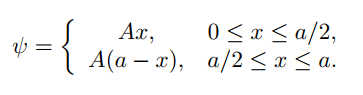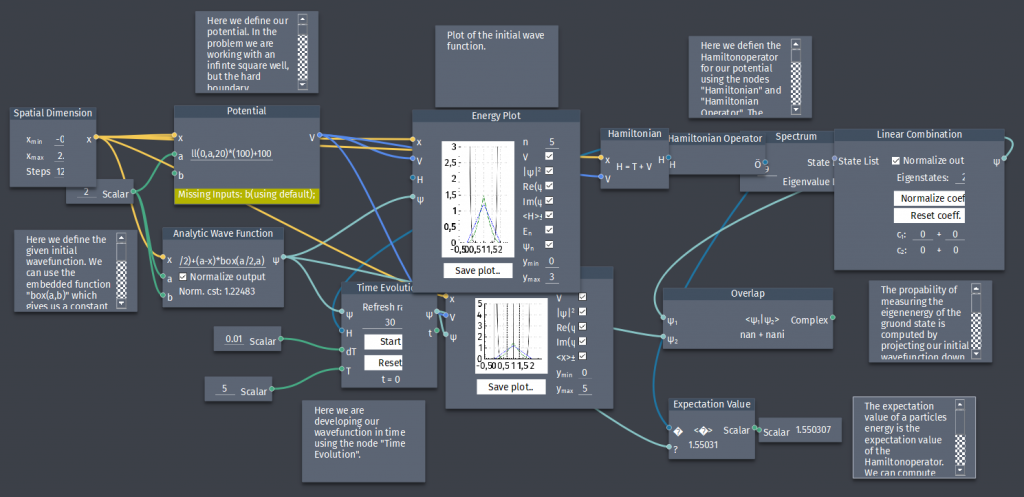# Infinite square well

A particle in the infinite square well has initial wavefunction:a) Plot $\Psi(x,0)$ and determine the constant $A$.

b) Find $\Psi(x,t)$.

c) What is the probability that a measurement of the energy would yield the ground state energy?

d) Find the expectation value of the energy.